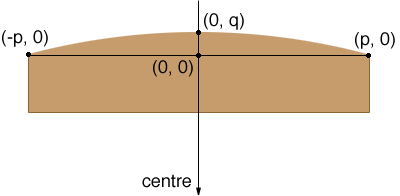SEARCH HOMEMath Central Quandaries & QueriesI am building an arched front cabinet that is 71 inches wide, 12 inches deep at both ends and 16 inches deep at the center. To accurately build this cabinet I need to known the radius of the circle that would form that arch. Thanks, JoeHi Joe,

Sue answered a similar question from Angela, the daughter of a welder, a while ago but she wanted much more than the radius of the circle. You can use Sue's response to find the radius but if you only need the radius there is a more immediate method.

Below is a diagram of your cabinet with some labels and coordinate axes. For your cabinet q = 4 inches and p = 71/2 = 35.5 inches. The centre of the circle is somewhere further down on the y-axis.Suppose the centre of the circle is at (0, y0) then the equation of the circle is

x2 + (y - y0)2 = r2

where r is the radius of the circle.

Since the circle passes through (o, q) and (p, 0),

(q - y0)2 = r2
p2 + y02 = r2

Thus

(q - y0)2 = p2 + y02

and simplification gives

y0 = (q2 - p2)/(2q)

From the diagram you can see that the radius of the circle is q plus the distance from the origin, (0, 0) to the centre of the circle, (0, y0). Since y0 is negative we drop the negative sign (using the absolute value function) and get

r = |y0| + q

For your cabinet with q = 4 inches and p = 35.5 inches I got

y0 = (q2 - p2)/(2q) = (42 - 35.52)/(24) = -155.5 inches

and

r = |y0| + q = |-155.5| + 4 = 159.5 inches.

PennyMath Central is supported by the University of Regina and The Pacific Institute for the Mathematical Sciences.